# Sequence Worksheets

#### How to Order Numbers in a Sequence

Number sequence refers to the placement of numbers either which number comes first or last. The consecutive movement of numbers in a series is called orders numbers in a sequence. There are two forms of number of orders that is ascending and descending order. Ascending order means stepping up the counting numbers or increase in order such as 225, 226, 227, 228, and 229. You can see how numbers are increasing and how they are moving forward. Similarly, descending order means stepping down the counting numbers in order such as 339, 338, 337, 336, and 335. In case of ascending order, numbers should be greater than one before it. In case of descending order sequence, every number is less than one that comes before it. We can also add and subtract all numbers in order of sequence. When you will add the number by moving one number to the next in an order. You can do this only in case of ascending order. When you will subtract the numbers by moving from one number to the upcoming one. You can do only in case of descending order. Similarly, you can multiply with rules for both forms of the orders of sequences.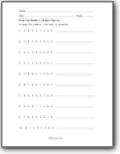###### List Them In Sequence

Take this string of single digit numbers and order them for least to greatest.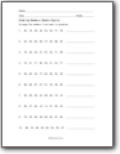###### Ordering Numbers (Double Digits)

Arrange the numbers from least to greatest.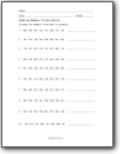###### Triple Digit Sequence

List these triple digit values in the proper sequence.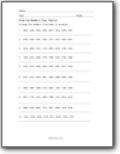###### Four Digits Values

Arrange the numbers from least to greatest.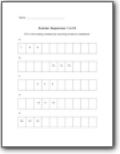###### Number Sequences 1 to 20

Fill in the missing numbers by counting forward or backward.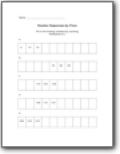###### Number Sequences by Fives

Fill in the missing numbers by counting forward by 5s.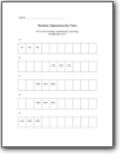###### Number Sequences by Tens

Fill in the missing numbers by counting forward by 10s.